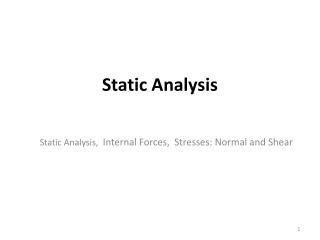DownloadDownload PresentationStatic Analysis

# Static Analysis

Télécharger la présentation## Static Analysis

- - - - - - - - - - - - - - - - - - - - - - - - - - - E N D - - - - - - - - - - - - - - - - - - - - - - - - - - -
##### Presentation Transcript

1. Static Analysis Static Analysis, Internal Forces, Stresses: Normal and Shear

2. Static Analysis Equation of equilibrium If only x-y plane

3. Types of Connection

4. Think! Lecture 2

5. FBD: Structure Discuss the approach? Lecture 1

6. ii) Equilibrium on Pin A i) Equilibrium on the whole system iv) Equilibrium on Pin D iii) Equilibrium on Pin C

7. Take Note of the tension and compression on the members

8. Two and three force body member Three-Force member

9. Two force member determination will set the direction of FAB Then, equilibrium equation will solve FBD: FAB, Cx and Cy

10. Find the forces on each member Discuss the approach?

11. Method 1: From Pin to Pin (equilibrium) Question: Can we solve the problem by treating the whole system as the initial FBD?

12. Discuss the approach? Use your engineering judgment? 1) What is the direction of the force at C? 2) If the downward force 0.75P is removed, which frame should be removed? Use your engineering judgment? Does length of frame has the effect on the force calculation? Lecture 1

13. Try! (FBD) Draw the FBD if P = 1500 N

14. Solution FBD = 5905.36 N Cx = 2952.68 N Cy= 3614.20 N C = 4666.98 N

15. Discuss the approach?

16. Internal Forces Considering the external and internal forces; the most critical is PBC = 30 kN. Therefore, by knowing the external and internal forces, we can decide the most critical part to be further analyzed.

17. Internal Forces Find the internal forces at point E Solve the FBD first m = 500 kg

18. solve FCD Solve Ax and Ay

19. Internal Forces solve ME Solve VE and NE Similar from Point B to E, apply the equilibrium equation to calculate the internal forces

20. Internal Forces: Example Example 1.1 Calculate the VC, NC and MC and their direction

21. Normal Stress

22. P: force that is perpendicular to plane ( the force is acted on the center of designated area) A: area of the plane Calculate the average normal stress: d = 200 mm Structure (Assume P = 100 kN ) Wide Flange Structure: W310 x 74 Angles L203 x 203 x 19.0

23. Allowable Normal Stress • The allowable stress is the maximum stress allowed due to the properties of the material. • Considered as SAFE • Considered as FAIL Safety Factor Safe F.S >= 1 Fail F.S < 1

24. Solve it A load W is supported by a 3‐cable system as shown in figure 1. The cable run along 2 pulleys F and D which is attached to the solid wall by a round beam EF and CD both of which are 30mm diameter and 300mm long. Ignore the mass of cable and beam. All cables are the same size. The sizes of the pulleys at D & F can be ignored. Gravity = 9.81m/s2 Answer the following questions: Find the internal stress at the mid point of round beam FE Calculate the normal stresses at cable AB, BD, BF Calculate the diameter of the cable is the allowable stress is 140 MPa